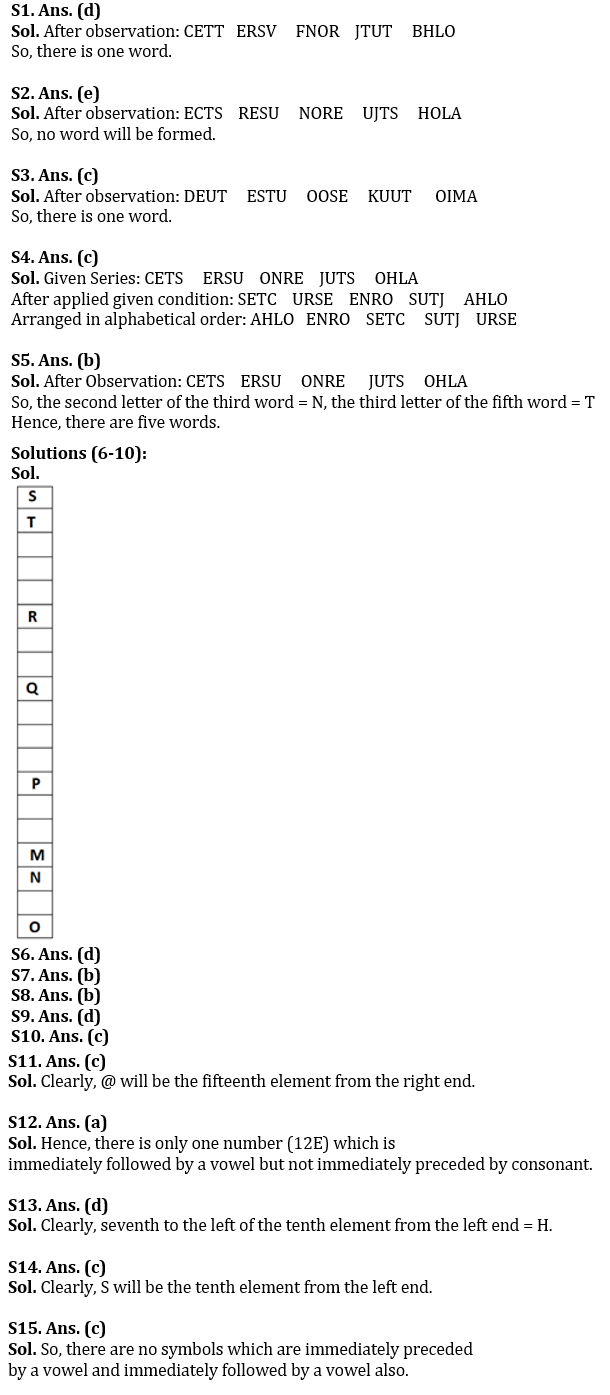Latest Banking jobs   »

# Reasoning Ability Quiz For Bank Foundation 2023- 16th January

Directions (1-5): Study the following data carefully and answer the questions accordingly.

CETS   ERSU   ONRE   JUTS   OHLA

Q1. If the last letter of each word is changed to its next letter in English alphabetical series and then the letters in each word are arranged from left to right according to the English alphabetical order within the word, then how many words will start with a vowel?
(a) Two
(b) More than three
(c) Three
(d) One
(e) None of these

Q2. If the first and the second letters of each word are interchanged then how many meaningful words will be formed?
(a) Three
(b) Four
(c) More than four
(d) Two
(e) None

Q3. If each consonant is changed to its next letter according to the English dictionary, then how many words will have more than two vowels?
(a) One
(b) Four
(c) Two
(d) Three
(e) None of these

Q4. If the first and the last letters of each word are interchanged and all the words are arranged according to English dictionary from left to right, then which of the following words will be third from the left end?
(a) ERSU
(b) OHLA
(c) CETS
(d) JUTS
(e) None can be formed

Q5. How many letters are there according to English alphabetical order between the second letter of the third word from the left end and the third letter of the fifth word from the right end?
(a) Seven
(b) Five
(c) Eight
(d) Six
(e) None of these

Directions (6-10): Study the following information carefully and answer the given questions.
A certain number of boxes are placed at one another such that one box is placed above another box in a stack. S is placed at the topmost position and just above T. R is placed at 4th position below T. O is placed at 3rd position below M. One box is placed between O and N. O is placed at the bottom. Two boxes are placed between R and Q. Minimum two boxes are placed between Q and T. Three boxes are placed between Q and P. Two boxes are placed between P and M.

Q6. If K is placed at 3rd position above P, then how many boxes are placed above K?
(a) Six
(b) Seven
(c) Eight
(d) Nine
(e) Ten

Q7. How many boxes are placed between N and Q?
(a) Five
(b) Seven
(c) Eight
(d) Six
(e) Ten

Q8. Which of the following box is placed just above N?
(a) P
(b) M
(c) O
(d) Q
(e) None of the above

Q9. How many boxes are placed in this stack?
(a) 17
(b) 16
(c) 18
(d) 19
(e) 20

Q10. Which of the following combination/s is/are correct?
(a) M – 15th position from top
(b) P – 12th position from top
(c) Q – 11th position from bottom
(d) N – 13th position from top
(e) All are correct

Directions (11-15): Study the following information carefully to answer the given questions:

Z O H M \$ 9 H 1 2 E & @ S U V 5 ¥ 3 R O O I T 4 3 % 4 \$ L 2 N E

Q11. If all the numbers are dropped in the above arrangement, then which will be the fifteenth element from the right end?
(a) U
(b) E
(c) @
(d) S
(e) None of these

Q12. How many such numbers are there in the above arrangement each of which is immediately followed by a vowel but not immediately preceded by consonant?
(a) One
(b) Two
(c) Three
(d) More than three
(e) None

Q13. Which of the following is the seventh to the left of the tenth element from the left end of the above arrangement?
(a) E
(b) @
(c) 2
(d) H
(e) 9

Q14. If all the symbols are dropped in the above arrangement, then which will be the tenth element from the left end?
(a) U
(b) R
(c) S
(d) H
(e) V

Q15. How many symbols are there which are immediately preceded by a vowel and immediately followed by a vowel also?
(a) One
(b) Two
(c) None
(d) Three
(e) None of these

Solutions## FAQs

### Q1. What is the direction of Point D with respect to Point Z?

Q1. What is the direction of Point D with respect to Point Z?

#### Congratulations!